# Investigation of Forced Convective and Subcooled Flow Boiling Heat Transfer Coefficients of Water-Ethanol Mixture: Numerical Study

Investigation of Forced Convective and Subcooled Flow Boiling Heat Transfer Coefficients of Water-Ethanol Mixture: Numerical Study
Department of Mechanical Engineering, B.M.S College of Engineering, Bull Temple Road, Bengaluru 560019, India

Department of Mechanical Engineering, National Institute of Technology Karnataka Surathkal, Mangalore, Karnataka 575025, India

Corresponding Author Email:
suhas_bg@yahoo.co.in
Page:
512-520
|
DOI:
https://doi.org/10.18280/ijht.390221
13 July 2019
|
Accepted:
14 December 2020
|
Published:
30 April 2021
| Citation

OPEN ACCESS

Abstract:

The subcooled flow boiling is related to the operation of electronic devices, Hybrid electric vehicle (HEV) Battery module and small catalytic reactors. It is well known that the operational temperature must be maintained to avoid any malfunction of these heat dissipative devices. In this paper the forced convective and subcooled flow boiling heat transfer coefficients of water-ethanol mixture is determined numerically by Volume of fluid analysis (VOF). The interaction between liquid and local vapour is analysed by solving the bubble volume of fraction in the numerical study. Crank Nicolson implicit scheme is used for discretizing the scalar convection equation for bubble void fraction and transforming into algebraic equation. Thomas Algorithm is used to solve the algebraic equations of bubble void fraction. The corrector predictor equation method is used to solve for bubble void fraction when the value obtained is less than 0 or exceeds 1. The thermodynamic and Thermophysical properties are substituted in the x-momentum and energy equation to determine the values of pressure drop, velocity and temperature of the fluid. From the temperature values, the subcooled flow boiling heat transfer coefficient is obtained. It is found that the addition of ethanol to water decreases the forced convective and subcooled flow boiling heat transfer coefficient of the water-ethanol mixture. The numerically determined heat transfer coefficient of water ethanol mixture is compared with that of the experimental results. The average deviation between the experimentally determined and numerically determined subcooled flow boiling heat transfer coefficient of water ethanol-mixture is found to be 24.13%.

Keywords:

bubble void fraction, numerical simulation, high speed camera, conventional channel, visualization

1. Introduction

The current technology in the nuclear industries, petroleum refineries, chemical industries, process industries, automobile industries, refrigeration systems etc. endeavor to meet the demand and supply in daily life of mankind. In these industries, heat is dissipated from the catalytic reactors, batteries, electronic devices, burners, radiators etc. The forced convective and subcooled flow boiling of water-ethanol mixture is pertinent to the operation of heat dissipative devices like small catalytic reactors, electronic devices and HEV battery module. The heat transfer coefficient of water-ethanol mixture is required to design cooling equipments for the heat dissipative devices [1-3]. During the past decades, only experimental studies to determine the heat transfer coefficient had been performed. But performing experiment will be tedious and therefore the alternative path is to determine by numerical methods. The experimental studies to date on the subcooled flow boiling have yielded only limited information on the basic two-phase hydrodynamic characteristics of water-ethanol mixture. Subcooled boiling is a kind of boiling that reaches the saturation temperature in the vicinity of a heated wall with the bulk temperature being below the wall temperature [4, 5]. On the other hand, benefit from the numerical method can be considered as alternative tool to provide useful physical information with limited cost. The numerical schemes for the prediction of two-phase flows can be classified into surface and volume methods [6-8]. Surface methods maintain sharp interfaces throughout the calculation. A disadvantage of these methods is that special treatment needs to be implemented to solve for interfaces which are exposed to large deformations. In volume methods, the different fluids are marked by an indicator function known as volume fraction or a level set [9-12]. The advantage of these methods is their ability to deal with arbitrarily shaped interfaces and to handle large deformations. The convective scalar transport is discretized for the bubble void fraction equations with a differencing scheme that guarantees physical values and thus preventing streaking of the transitional area over the grids [13, 14]. Bubble void fraction is the presence of bubble in the subcooled fluid and its presence affects the thermo physical and thermodynamic properties of the fluid.

Detailed investigation on subcooled flow boiling of water-ethanol mixture is limited in literature which is essential to design the cooling devices. In view of this, the understanding of the bubble behavior and its effect on heat transfer significantly contributes to a better understanding of physical phenomena in subcooled flow boiling [15, 16]. Hence flow visualization is essential to study the bubble dynamics of water-ethanol mixture during subcooled flow boiling. The experimental studies on the flow boiling have shown limited information on the basic two-phase hydrodynamic characteristics of binary mixtures. The benefit from the recent studies on numerical analysis can be considered as an alternative path to provide useful physical information with limited cost. However, the detailed literature shows that the numerical analysis for determining the forced convective and subcooled flow boiling heat transfer coefficients for water-ethanol mixtures are not available. The two-fluid model essentially demands momentum equation for each of the phases. The interfacial transport of momentum and heat depends on the relations derived from the empirical equations and are not accurate till the date . In view of the great significance of the reliable data for water-ethanol mixture as an alternative coolant, an independent study is undertaken here to determine the subcooled flow boiling characteristics of this mixture.

The present work consists of determining the forced convective and subcooled flow boiling heat transfer coefficients of water ethanol mixture. Heat transfer related to forced convection and subcooled flow boiling of water-ethanol mixture is determined from mathematical modelling and numerical simulation. The Thermophysical and thermodynamic properties of the subcooled boiling fluid of water-ethanol mixture are determined from mathematical modelling. The bubble void fraction is determined and substituted in the mixing rule equation of thermodynamic and Thermophysical properties. The thermodynamic and Thermophysical properties are substituted in the x-momentum and energy equation to determine the values of pressure drop, velocity and temperature of the fluid. From the temperature values, the subcooled flow boiling heat transfer coefficient is obtained and compared with that of experimental results.

2. Methodology

The schematic diagram of the experimental test set up is shown in Figure 1. The photographic images of the experimental set up are shown in Figure 2(a) and 2(b).

## 1.jpg(1) Rectangular aluminum block consisting of two rectangular channels (2) Condenser coil dipped in ice water bath (3) Reservoir (4) Peristaltic pump (5) Preheater (6) Cartridge heaters (7) Thermocouples to measure wall temperature (8) Thermocouple to measure fluid inlet temperature (9) Thermocouple to measure fluid outlet temperature (10) Temperature indicator panel (11) High speed camera (12) Light source (13) Data Acquisition system for flow visualization

Figure 1. Schematic diagram of experimental setup

The experimental set up is a closed loop having a rectangular aluminum block consisting of two rectangular channels, condenser coil dipped in ice water bath, reservoir, preheater and pump having variable flow rate. The aluminum block consisting of two channels of 10 mm (width) × 10 mm (height) × 150 mm (Length). The two cartridge heaters are inserted inside the aluminum block. The aluminum block is considered as cold plate. Heat loss is prevented by providing mineral wool as insulating material. The wall temperature, the fluid inlet and outlet temperatures of the channel are measured by thermocouples which are displayed on the temperature indicator panel. Temperature of bottom wall of channel is determined by temperature gradient between the first row and second row of thermocouples which are arranged in aluminum block as shown in Figure 3. Flow visualization is achieved with the help of high speed camera. The procedure followed during the flow visualization is provided in Figure 4. Lab view vision builder software is used for image processing.

## 1a.jpg(1) High speed camera (2) Condenser coil dipped in ice water bath (3) Reservoir (4) Peristaltic pump (5) Preheater (6) Temperature indicator panel (7) Light source

(a) Front view of the experimental setup

## 1b.jpg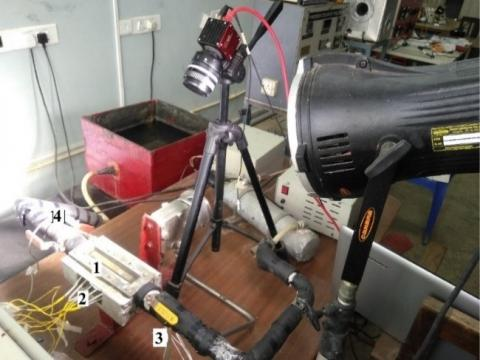(1) Rectangular aluminum block consisting of two rectangular channels (2) Thermocouples to measure wall temperature (3) Thermocouple to measure channel inlet temperature (4) Thermocouple to measure outlet fluid temperature

(b) Rear view of the experimental setup

Figure 2. Photographic image of experimental test set up

Specifications of the equipment used in the experimental test set up are shown in Table 1 and Table 2 [18-20].

Figure 5 shows the method to measure contact angle. The angle between the tangent and the channel wall is measured as the contact angle. The width of the channel is considered as the reference to determine the diameter of the bubble. Camera is placed as shown in Figure 6. However, it is found that there is no magnificent variation in measuring the diameter of the bubble as the bubbles are considered as sphere.

## 3.jpg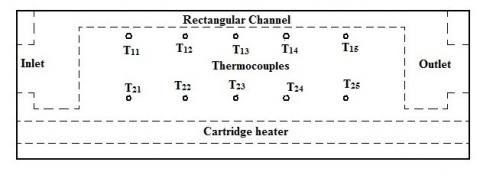Figure 3. Arrangement of thermocouples in the cold plate

## 4.jpg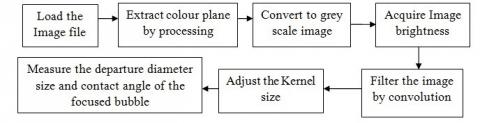Figure 4. Image processing

## 5.jpg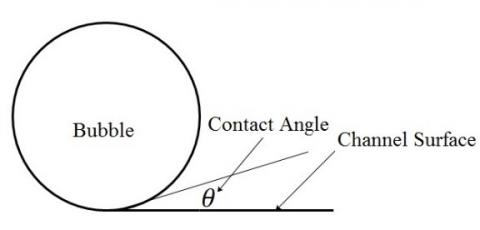Figure 5. Contact angle

## 6.jpg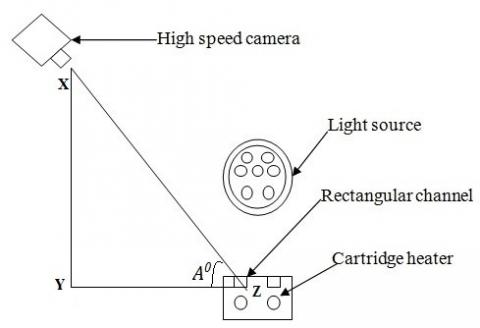Figure 6. High speed camera and the channel

From the trigonometric relation $A^{\circ}$ is determined.

$\frac{X Y}{Y Z}=\tan \left(A^{\circ}\right)$

$A^{\circ}$ is the correction factor needed to measure the actual contact angle. XY is the distance from the lens of the camera the camera stand (Y) which considered as parallel to the wall of channel. YZ is the distance from the wall of the channel to the point on the camera stand.

$A^{\circ}=\tan ^{-1}\left(\frac{X Y}{Y Z}\right)$

$\frac{\text { Measured contact angle }}{\text { Actual contact angle }}=\frac{X Z}{Y Z}=\frac{X Z}{X Z \cos \left(A^{\circ}\right)}$

Actual contact angle $=$ Measured contact angle $\times \cos \left(\mathrm{A}^{\circ}\right)$

Measured contact angle is considered from the image processing.

Table 1. Instruments and equipments used in the present experiment

 Instruments/Equipments Specifications k-type thermocouples (12 no’s) Range: -20℃ to 400℃, Sheath length: 20 mm, sheath diameter: 1.2 mm Cartridge heater (2 no’s) Diameter: 12.7 mm, Length: 180 mm, capacity: 750 W Peristaltic pump Capacity: 100 liters per hour, Operating pressure: Atmospheric Preheater Chamber capacity: 4 Liters, Heater capacity: 3 kW. High speed camera AOS Promon 501, 1459 frames per second, 480×240 (resolutions)

Table 2. Specifications of high speed camera and source light

 Processor AOS Promon 501 Lens 50 mm Aperture setting f/1.4 D Shutter speed 1/15 Frames per second 1459 Resolution 480×240 pixels LED PAR Light Slim die cast body, Power 120W, beam 25 degree, CRI>85, DMX 512 Auto, sound active, 3 section lightweight aluminum stand
3. Numerical Analysis

3.1 Forced convective heat transfer coefficient: Mathematical modelling

The continuity equation is represented by Eq. (1)

$\frac{\partial u}{\partial x}=0$    (1)

The x-momentum-Navier Stokes equation is represented by Eq. (2).

$\frac{\partial u}{\partial t}=-\frac{\partial p}{\partial x}+\mu\left(\frac{\partial^{2} u}{\partial y^{2}}\right)$      (2)

The energy equation is represented by Eq. (3).

$C_{p} \frac{\partial T}{\partial t}=-u \frac{d p}{d x}+k \frac{\partial^{2} T}{\partial x^{2}}+k \frac{\partial^{2} T}{\partial y^{2}}+\mu\left(\frac{d u}{d y}\right)^{2} v$     (3)

The wall temperature determined from the experiment is considered as the Dirichlet boundary condition. The pressure drop and velocity in the x-momentum equation are determined by pressure correction method. The determined values of pressure drop and velocity are substituted in the energy equation and solved by the Lax Wandroff method to determine the temperature of the fluid. The forced convective heat transfer coefficient is determined from the known values of wall temperature, heat flux and determined values of fluid temperature as shown in Eq. (4).

$h=\frac{q^{\prime \prime}}{\left(T_{W}-T_{f}\right)}$     (4)

Specific heat of the mixture is calculated using simple mixing rule. Thermal conductivity and liquid viscosity are calculated by Flippov and McLaughlin Equation [21, 22] represented in the Eq. (5) and Eq. (6).

$\frac{k_{m}-k_{i}}{k_{j}-k_{i}}=C m_{f j}^{2}-m_{f i}(1-C)$     (5)

$\ln \left(\mu_{m}\right)=x_{i} \ln \mu_{i}+x_{j} \ln \mu_{j}$    (6)

Volume of fluid method (VOF) is adopted in the present analysis. In this analysis, the thermophysical and thermodynamic properties of the subcooled boiling fluid are determined by incorporating bubble void fraction (α) by simple mixture rule. The thermodynamic and thermophysical properties are substituted in the x-momentum and energy equation to determine the values of pressure drop, velocity and temperature of the fluid. From the temperature values, the subcooled flow boiling heat transfer coefficient is determined and compared with that of experimental results. The code is developed by using Matlab R 2018 programming to solve for heat transfer coefficient. The sample image of bubble formation of water at heat flux=90.4 kW/m2, mass flux=76.67 kg/m2-s and inlet temperature=303 K is shown in Figure 7. This sample is selected from the experiment. The channel containing the bubbles are divided into suitable grids to calculate the bubble void fraction. The conservative form of scalar convection equation for the bubble void fraction is given by Eq. (7).

$\frac{\partial \alpha}{\partial t}=u \frac{\partial \alpha}{\partial x}$    (7)

The Crank Nicolson implicit scheme is used as technique for discretization as shown in Eq. (8).

$\frac{\alpha_{i}^{t+1}-\alpha_{i}^{t}}{\Delta t}=u_{i n}\left\{\frac{\left(\frac{\alpha_{i+1}^{t+1}+\alpha_{i+1}^{t}}{2}\right)-\left(\frac{\alpha_{i}^{t+1}+\alpha_{i}^{t}}{2}\right)}{\left(\frac{\Delta x}{2}\right)}\right\}$     (8)

## 7.png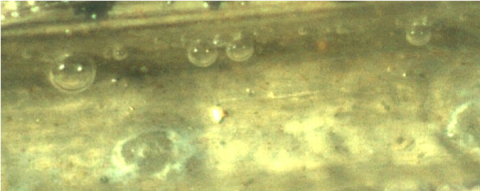Figure 7. Bubble formation of water at Heat flux=90.4 kW/m2 and mass flux=76.67 kg/m2-s

Algebraic equations are obtained from Eq. (6) is solved to determine bubble void fraction by using Tridiagonal Matrix Algorithm (TDMA). The bubble void fraction is solved in the control surface as upwind, donor and accepter cells with the face values in between the interfaces of grid as shown in Figure 8. Initially the values are assumed suitably varying from 0 to 1, depending upon occupation of bubbles in the channel surface. The bubble void fraction with values more than one and less than zero are obtained. Hence the corrector-predictor steps are involved to solve these void fractions. The new amount of fluid to be convected over the face is determined by subtracting the unboundedness error from the original amount of fluid convected over the face .

## 8.jpg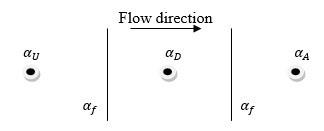Figure 8. Control area to solve bubble void fraction

3.1.1 Prediction of $\alpha$ in grid centre by corrector predictor method

Following steps are followed when $\alpha_{D}$ values obtained exceed 1 or negative.

$\beta_{f}=\left\{\min \left\{\frac{\cos \left(2 \theta_{f}+1\right)}{2}\right\}, 1.0\right\}$     (9)

$\beta_{f}$ is called weighing factor. The weighing factor incorporates the unboundedness error while calculating the bubble void fraction at the grid center and the face.

$\Delta \alpha=\frac{\alpha_{A}{ }^{t}+\alpha_{A}{ }^{t+1}}{2}-\frac{\alpha_{D}{ }^{t}+\alpha_{D}{ }^{t+1}}{2}$    (10)

$\beta_{f}^{\prime}=\min \frac{E^{-}\left(2+C_{f}-2 C_{f} \beta_{f}\right)}{2 C_{f}\left(\Delta \alpha^{*}-E^{-}\right)}, \beta_{f} \quad$ When $\Delta \alpha^{*}>E^{-}$    (11)

$\beta_{f}^{\prime}=0$, When $\Delta \alpha^{*}<E^{-}$    (12)

$E^{-}=\max \left\{-\alpha_{\mathrm{D}}^{\mathrm{t}+\delta \mathrm{t}}, 0\right\}$ When $\alpha_{D}^{t+1}<0$     (13)

$E^{+}=\max \left\{\alpha_{\mathrm{D}}^{\mathrm{t}+\delta \mathrm{t}}-1,0\right\}$ When $\alpha_{D}^{t+1}>1$    (14)

$\beta_{f}^{\prime}=\min \frac{E^{-}\left(2+C_{f}-2 C_{f} \beta_{f}\right)}{2 C_{f}\left(-\Delta \alpha^{*}-E^{+}\right)}, \beta_{f}$ When $-\Delta \alpha^{*}>E^{+}$     (15)

$\beta_{f}^{\prime}=0$, When $-\Delta \alpha^{*}<E^{+}$    (16)

$\beta_{f}^{*}=\beta_{f} \sim \beta_{f}^{\prime}$    (17)

The corrected weighting factor $\beta_{f}^{*}$ should be always less than or equal to the previous weighting factor. Otherwise, the contribution of the downwind cell starts to increase and so also the degree of unboundedness increases. The lower limit on $\beta_{f}^{*}$ remains zero and this is applied to Eq. (18) to obtain bounds for $\beta_{f}^{\prime}$.

$\alpha^{* *}{ }_{f}=\left(1-\beta_{f}^{*}\right)\left[\frac{\alpha_{D}^{t}+\alpha_{D}^{t+\delta t}+E^{-}}{2}\right]+\left[\frac{\alpha_{A}^{t}+\alpha_{A}^{t+\delta t}+E^{-}}{2}\right]$    (18)

Or

$\alpha_{f}^{* *}=\left(1-\beta_{f}^{*}\right)\left[\frac{\alpha_{D}^{t}+\alpha_{D}^{t+\delta t}+E^{+}}{2}\right]+\left[\frac{\alpha_{A}^{t}+\alpha_{A}^{t+\delta t}+E^{+}}{2}\right]$   (19)

where, $\alpha_{f}^{* *}$ is the new face value and and $E^{-}$ and $E^{+}$ the magnitude of the unbounded void value.

$\alpha_{f}^{*}=\alpha_{f}^{* *} \pm \frac{E^{-}}{c}$     (20)

Or

$\alpha_{f}^{*}=\alpha_{f}^{* *} \pm \frac{E^{+}}{c}$    (21)

$\alpha_{f}^{\prime \prime}=\left(1-\beta_{f}\right) \frac{\alpha_{D}^{t}+\alpha_{D}^{t+\delta t}}{2}+\beta_{f} \frac{\alpha_{A}^{t}+\alpha_{A}^{t+\delta t}}{2}$    (22)

Eq. (23) is used for calculating $\alpha_{D}$ for next time step

$\alpha_{D}^{c}=\frac{2 \alpha_{f}^{*}+\beta_{f} \alpha_{D}^{t}-\alpha_{D}^{t}-\beta_{f} \alpha_{A}^{t}-\beta_{f} \alpha_{A}^{t+\Delta t}}{\left(1-\beta_{f}\right)}$     (23)

3.1.2 Prediction of $\alpha$ in face centre by corrector predictor method

Following steps are followed when $\alpha_{f}$ (face values) obtained exceed 1 or negative.

$\widetilde{\alpha_{D}}=\frac{\alpha_{D-\alpha_{U}}}{\alpha_{A}-\alpha_{I I}}$      (24)

$\widetilde{\alpha_{f}}=\frac{\alpha_{f}-\alpha_{U}}{\alpha_{A}-\alpha_{U}}$     (25)

$\tilde{\alpha}_{f C B C}=\min \left\{\frac{\widetilde{\alpha_{D}}}{c}, 1.0\right\}$ When $0 \leq \tilde{\alpha}_{D} \leq 1$     (26)

$\tilde{\alpha}_{f C B C}=\widetilde{\alpha_{D}}$ When $\tilde{\alpha}_{D}<0, \widetilde{\alpha_{D}}>1$    (27)

$\widetilde{\alpha_{f U Q}}=\min \left\{\frac{8 c{\widetilde{\alpha}}_{D}+(1-c)\left(6 \widetilde{\alpha_{D}{+3}}\right)}{8}\right\}$ When $0 \leq \tilde{\alpha}_{D} \leq 1$    (28)

$\widetilde{\alpha_{f U Q}}=\tilde{\alpha}_{D}$ When $\tilde{\alpha}_{D}<0, \widetilde{\alpha_{D}}>1$    (29)

The above derivation for the volume of fluid method is a resolution scheme to be carried out only in one-dimension.

$\alpha_{f}^{c}=\beta_{f} \tilde{\alpha}_{f C B C}+\left(1-\beta_{f}\right) \tilde{\alpha}_{f U Q}$    (30)

where, $\tilde{\alpha}_{f B C}$ is bubble void fraction in face center to satisfy boundedness criteria. $\tilde{\alpha}_{\text {fQuick }}$ is bubble void fraction in face center to satisfy conservative criteria by QUICK scheme.  When  is more than 1 or less than 0 in the grid face and grid centre, the average values obtained from Eq. (28) and Eq. (36) are considered for the new value at the grid face.

3.1.3 Mixture rule

The viscosity, specific heat and thermal conductivity are determined by simple mixture rule as given by Eqns. (31), (32) and (33).

$\mu=\alpha \mu_{l m}+(1-\alpha) \mu_{v m}$     (31)

$k=\alpha k_{l m}+(1-\alpha) k_{v m}$      (32)

$C_{p}=\alpha C_{p l m}+(1-\alpha) C_{p v m}$    (33)

The pressure drop and velocity in the Eq. (2) are determined by pressure correction method. The values of pressure drop and velocity are substituted in the Eq. (3) and solved by the Lax Wandroff method to determine the temperature of the fluid. The subcooled flow boiling heat transfer coefficient is determined from the known values of wall temperature, heat flux and determined values of fluid temperature by Eq. (4).

3.1.4 Pressure correction technique and Lax Wandroff Explicit scheme

The procedure to solve the momentum and energy equations by using Pressure correction method and Lax Wandroff Explicit method is discussed in detail in the literature .

3.2 Grid independent study

The pressure drop, velocity and temperature are calculated by numerical techniques such as pressure correction method and the Lax Wandroff explicit method for mathematical modelling. The grid independence for the pressure drop of water at heat flux=90.4 kW/m2 and mass flux=76.67 kg/m2-s and inlet temperature=303 K is checked. The change in pressure drop and temperature of water are not very significant. The grid size of 15 X 8 are chosen in the present study. During the numerical study, it is found that there is no significant variation in the single phase heat transfer coefficient with grid numbers.

4. Results and Discussions

The variation of heat transfer coefficients along the length of the channel for water are shown in Figure 9 (a) and (b). The variation of heat transfer coefficients of water-ethanol mixture of ethanol volume fraction 25% is shown in Figure 9 (c). It can be seen that the heat transfer coefficient decreases along the length of the channel. This is due to the increase in thermal boundary layer thickness along the length of the channel. The results obtained from pressure correction SIMPLE solution method by ANSYS are validated with results obtained from mathematical modelling and experiment.

## 9a.jpg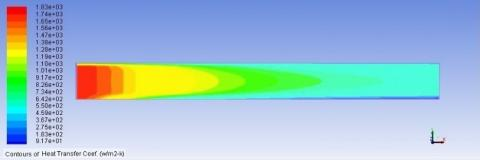(a) Variation of forced convective heat transfer coefficient along the length of the channel for water at heat flux =21.78 kW/m2 and mass flux=76.67 kg/m2-s

## 9b.jpg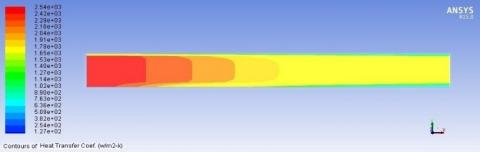(b) Variation of forced convective heat transfer coefficient along the length of the channel for water at heat flux =21.78 kW/m2 and mass flux=228.33 kg/m2-s

## 9c.jpg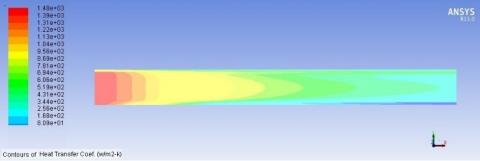(c) Variation of forced convective heat transfer coefficient along the length of the channel for water-ethanol mixture of ethanol volume fraction 25% at heat flux =21.78 kW/m2 and mass flux=228.33 kg/m2-s

Figure 9. Heat transfer coefficient

The validation of single phase forced convective heat transfer coefficient of water with mass flux is shown in Figure 10. It is found that the forced convective heat transfer coefficient of water obtained from numerical simulation deviated by 17.1% from that of the experiment and 21.3% from that of mathematical modelling.

## 10.jpgFigure 10. Validation of single phase forced convective heat transfer coefficient of water at heat flux=21.78 kW/m2

The deviation is due to certain assumptions made during the numerical simulation and mathematical modelling.

1. Single channel analysis is carried out for both the numerical and mathematical modelling.
2. The momentum and energy equations are simplified to obtain one dimensional flow for mathematical modelling.
3. Uniform wall temperature boundary condition is assumed at the channel wall surface.
4. The change in heated wall temperature does not change with time steps.
5. Thermodynamic and Thermophysical properties are assumed to be constant with change in time steps.

Mixed type boundary conditions are adopted to solve numerically, wherein the experimentally determined wall temperature and wall heat flux are specified. No slip boundary condition and wall temperature (Dirichlet boundary condition) is specified to determine the velocity, pressure and fluid temperature by mathematical modelling. Unlike the experiment, in mathematical modelling and numerical simulation, the heat flux and wall temperature specified are considered to be constant. Figure 11 shows the variation of single phase forced convective heat transfer coefficient with water-ethanol mixture of different ethanol volume fraction. The heat transfer coefficient decreases with increase in ethanol volume fraction because of lower thermal conductivity and thermal capacity of ethanol than that of water. In all the figures, it can be seen that that under the same heat flux and different mass flux, the forced convection heat transfer coefficient of water varies with the change of channel length. This may be attributed to the thermal boundary layer thickness. At the inlet of the channel, thermal boundary layer is lesser which causes an increase in heat transfer coefficient. Thermal boundary layer increases when the fluid passes over the channel and thus causing decrease in heat transfer coefficient.

The variation of bubble void fraction and heat transfer coefficients of mixtures with different ethanol volume fractions are shown in Figure 12. Out of 1459 frames 15 frames are considered to solve for bubble void fraction and heat transfer coefficients. This procedure is followed for water ethanol mixtures of different ethanol volume fractions (25%, 50%, 75% and 100%). It can be seen that bubble void fraction and subcooled flow boiling heat transfer coefficient decreases with increase in ethanol volume fraction. The bubble void fraction is obtained from the bubbles that are formed on the surface. The bubble void fraction is determined from the still images at various frames. The still images depict the bubbles which are attached to the wall surface. Initially heat transfer coefficient decreases with the bubble formation. This is because the bubble acts as a vapour blanket and thus prevents the heat transfer from the wall to the surrounding liquid. But it is seen that when the bubble departs from the surface the heat transfer coefficient increases [25-27]. The bubble condenses into the subcooled part of the flowing liquid and acts as an energy carrier and thus increases the heat transfer coefficient. The formation of bubbles decreases with increase in ethanol volume fraction.

## 11.jpg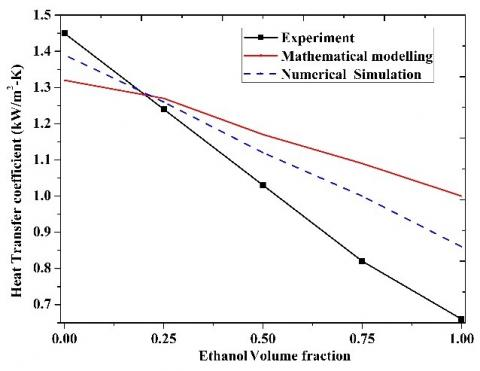Figure 11. Variation of single phase forced convective heat transfer coefficient with ethanol volume fraction at heat flux=21.78 kW/m2

Variation of heat transfer coefficient with ethanol volume fraction is shown in Figure 13. The numerically determined heat transfer coefficient decreases with addition of ethanol to water. The average deviation of 24.13 % is observed for subcooled flow boiling heat transfer coefficient of water-ethanol mixture when compared with that of experiment. The reason being that, only the bubble void fraction is determined and substituted in the thermophysical and thermodynamic properties and effect of Marangoni convection is not considered. The Marangoni convection is the surface tension between the liquid and vapor interphases. The deviations are observed due to following assumptions.

1. Flow is assumed to be one dimensional, hence change in velocity in the axial direction is negligible. Velocity changes only along normal direction as per law of velocity profile.
2. Effect of nucleation and hydrodynamic instabilities during the subcooled flow boiling are not considered in the simulation.
3. Uniform wall temperature boundary condition is assumed at the channel wall surface. The change in heated wall temperature does not change with time steps.
4. The thermodynamic and thermophysical properties are obtained from bubble void fraction from the mixture rule. These properties are assumed to be constant with change in time steps.
5. Effect of vapour generation rate, forces due to lift, drag and dispersion is not incorporated in the x-momentum and energy equations because the heat transfer coefficient is determined from the bubble volume of fraction which are obtained from still images.
6. Mixture composition vapour phase is assumed to be constant and same as liquid phase composition.

## 12.jpg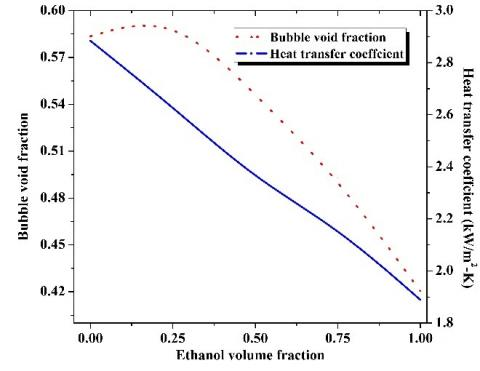Figure 12. Variation of subcooled flow boiling heat transfer coefficient and bubble void fraction with ethanol volume fraction

## 13.jpgFigure 13. Comparison of numerical result with that of the experiment at heat flux=90.4 kW/m2

5. Conclusions and Scope for Future Work

The photographic images of bubbles obtained using high speed camera are used to determine the bubble void fractions. The interaction between liquid and local vapour is analysed by solving the bubble volume of fraction in the numerical study. This bubble volume of fraction is considered in the momentum and energy equations to determine the heat transfer coefficient of the binary mixture. The values obtained are compared with that of experiment. Following conclusions are arrived from the present work.

• It is observed that the experimentally determined subcooled flow boiling heat transfer coefficient increases with the addition of ethanol to water initially upto 25% ethanol volume fraction, but at 50% and 75% ethanol volume fractions the heat transfer coefficient reduces. The pure ethanol has marginally higher value than the mixture of ethanol volume fraction 75%.
• From the numerical analysis it is concluded that the addition of ethanol to water decreases the forced convective and subcooled flow boiling heat transfer coefficient of the water-ethanol mixture.
• It is found that the forced convective heat transfer coefficient of water obtained from numerical simulation deviated by 17.1% from that of the experiment and 21.3 % from that of mathematical modelling.
• The average deviation between the experimentally determined and numerically determined subcooled flow boiling heat transfer coefficient of water ethanol-mixture is 24.13%.

The possible future research can be carried out experimentally and numerically as follows:

• The present experiment may be extended upto critical heat flux to determine the heat transfer coefficient in the different regimes of boiling.
• The experiment can be conducted with different binary mixtures. Repeating these experiments with different binary mixtures for a wide range of aspect ratios would further expand understanding the channel geometries which influences the bubble departure.
• The forces acting on the bubbles during subcooled boiling liquid in low-aspect ratio and microchannels may be identified.
• The recently discovered confinement pressure effects deserve extensive experimentation to determine the degree to which these effects influence the flows. Such studies should attempt to demonstrate the additional nucleation due to bubble induced water hammer propagation and add to the dataset of bubble growth rates for a broader range of channels cross-sections and bubble pressures.
• The development of fiber optic sensors will further extend the experimental parameter set to include slug velocity, size, and growth rates as well as allow the first maps in time and space of liquid temperature and void fraction. Such maps would be extremely valuable in understanding the flow instabilities during the boiling.
Acknowledgement

Authors wholeheartedly express deep gratitude towards the management of B.M.S College of Engineering and NIT Surathkal for their support to the research work.

Nomenclature
 c Courant number h Heat transfer coefficient (kW/m2-K) p Pressure (Bar) Re Reynolds number q” Heat flux (kW/m2) t Time T Temperature (K) u Liquid velocity in parallel direction (m/s) u* Liquid velocity of previous iteration (m/s) u’ Corrected liquid velocity (m/s) x Vapour fraction X Position (m) We Weber numbe Greek symbols $\alpha$ Bubble void fraction $\alpha_{A}$ Bubble void fraction in accepter cell $\alpha_{D}$ Bubble void fraction in donor cell $\widetilde{\alpha_{D}}$ Normalized bubble void fraction in donor cell $\alpha_{f}^{* *}$ New value of bubble void fraction in face cell $\widetilde{\alpha_{f}}$ Normalized bubble void fraction in face cell $\widetilde{\alpha_{f B C}}$ Boundedness criteria for bubble void fraction in face cell $\widetilde{\alpha_{{fquick }}}$ QUICK scheme for bubble void fraction in face cell $\alpha_{f}^{c}$ Corrected value of Bubble void fraction in face cell $\beta_{f}$ Weighing factor $\beta_{f}^{*}$ New value of weighing factor $c_{f}$ Cell Courant number $\Delta p_{\text {Sat }}$ Difference between the saturated pressure and vapour pressure (Bar) $\Delta p^{\prime}$ Corrected pressure drop (Bar) $\Delta p^{*}$ Pressure drop of previous iteration (Bar) $\Delta T$ Temperature difference (K) $\rho$ Density (kg/m3) $\mu$ Dynamic viscosity (kg/m-s) Subscripts BC Boundedness criteria l Liquid lm Mixture in liquid phase QUICK Quadratic ultimate implicit convection kinetics vm Mixture in vapour phase
References

 Suhas, B.G., Sathyabhama, A. (2018). Experimental study on forced convective and subcooled flow boiling heat transfer coefficient of water-ethanol mixtures: An application in cooling of HEV battery module. Heat and Mass Transfer, 54: 277-290. https://doi.org/10.1007/s00231-017-2122-4

 Hasanpour, B., Irandoost, M.S., Hassani, M., Kouhikamali, R. (2018). Numerical investigation of saturated upward flow boiling of water in a vertical tube using VOF model: Effect of different boundary conditions. Heat and Mass Transfer, 54(7): 1925-1936. https://doi.org/10.1007/s00231-018-2289-3

 Pothukuchi, H., Kelm, S., Patnaik, B.S.V., Prasad, B.V.S.S.S., Allelein, H.J. (2018). Numerical investigation of subcooled flow boiling in an annulus under the influence of eccentricity. Applied Thermal Engineering, 129: 1604-1617. https://doi.org/10.1016/j.applthermaleng.2017.10.105

 Azizifar, S., Ameri, M., Behroyan, I. (2020). Subcooled flow boiling of water in a metal-foam tube: An experimental study. International Communications in Heat and Mass Transfer, 118: 104897. https://doi.org/10.1016/j.icheatmasstransfer.2020.104897

 Walunj, A., Sathyabhama, A. (2019). Experimental investigation on transient pool boiling heat transfer from rough surface and heat transfer correlations. International Journal of Heat and Technology, 37(2): 545-554. https://doi.org/10.18280/ijht.370223

 Sussman, M., Puckett, E.G. (2000). A coupled level set and volume-of-fluid method for computing 3D and axisymmetric incompressible two-phase flows. Journal of Computational Physics, 162(2): 301-337. https://doi.org/10.1006/jcph.2000.6537

 Gueyffier, D., Li, J., Nadim, A., Scardovelli, R., Zaleski, S. (1999). Volume-of-fluid interface tracking with smoothed surface stress methods for three-dimensional flows. Journal of Computational Physics, 152(2): 423-456. https://doi.org/10.1006/jcph.1998.6168

 Gerlach, D. (2006). Comparison of volume-of-fluid methods for surface tension-dominant two-phase flows. International Journal of Heat and Mass Transfer, 49(3): 740-754. https://doi.org/10.1016/j.ijheatmasstransfer.2005.07.045

 Welch, S.W.J., Wilson, J. (2000). A volume of fluid based method for fluid flows with phase change. Journal of Computational Physics, 160(2): 662-682. https://doi.org/10.1006/jcph.2000.6481

 Kunkelmann, C., Stephan, P. (2009). CFD simulation of boiling flows using the volume-of-fluid method within Open FOAM. Numerical Heat Transfer, Part A: Applications, 56(8): 631-646. https://doi.org/10.1080/10407780903423908

 Yuan, M.H. (2008). Numerical simulation of film boiling on a sphere with a volume of fluid interface tracking method. International Journal of Heat and Mass Transfer 51(7): 1646-1657. https://doi.org/10.1016/j.ijheatmasstransfer.2007.07.037

 Son, G., Dhir, V.K. (1998). Numerical simulation of film boiling near critical pressures with a level set method. Transactions-ASME, 120: 183-192. https://doi.org/10.1115/1.2830042

 Wu, J.F., Dhir, V.K., Qian, J.L. (2007). Numerical simulation of subcooled nucleate boiling by coupling level-set method with moving-mesh method. Numerical Heat Transfer, Part B: Fundamentals, 51(6): 535-563. https://doi.org/10.1080/10407790601177763

 Wacławczyk, T., Koronowicz, T. (2008). Comparison of CICSAM and HRIC high-resolution schemes for interface capturing. Journal of Theoretical and Applied Mechanics, 46(2): 325-345.

 Suhas, B.G., Sathyabhama, A. (2017). Bubble dynamics of water-ethanol mixture during subcooled flow boiling in a conventional channel. Applied Thermal Engineering, 113: 1594-1608. https://doi.org/10.1016/j.applthermaleng.2016.11.126

 Bhati, J., Paruya, S. (2021). Numerical simulation of bubble dynamics in subcooled flow boiling in a channel. Nuclear Engineering and Design, 371: 110945. https://doi.org/10.1016/j.nucengdes.2020.110945

 Paramanantham, S.S., Ha, C.T., Park, W.G. (2018). Numerical investigation of single and multiple bubble condensing behaviors in subcooled flow boiling based on homogeneous mixture model. International Journal of Mechanical Sciences, 136: 220-233. https://doi.org/10.1016/j.ijmecsci.2017.12.040

 Suhas, B.G., Sathyabhama, A. (2017). Experimental investigation of heat transfer coefficient and correlation development for subcooled flow boiling of water–ethanol mixture in conventional channel. Journal of Thermal Science and Engineering Application, ASME, 9(4): 041003-11. https://doi.org/10.1115/1.4036202

 Suhas, B.G., Sathyabhama, A. (2018). Heat transfer and force balance approaches in bubble dynamic study during subcooled flow boiling of water–ethanol mixture. Experimental Heat Transfer, Taylor and Francis, 31(1): 1-21. https://doi.org/10.1080/08916152.2017.1328469

 Suhas, B.G., Sathyabhama, A., Veerabhadrappa, K., Suresh Kumar, U., Kiran Kumar, U. (2019). Wall heat flux partitioning analysis for subcooled flow boiling of water-ethanol mixture in conventional channel. Frontiers in Heat and Mass Transfer (FHMT), 13. http://dx.doi.org/10.5098/hmt.13.16

 Flippov, L.P. (1968). Research of liquid thermal conductivity at Moscow university. Int. J. of Heat Mass Transfer, 11: 331-345.

 Ratcliff, G.A., Khan, M.A. (1971). Prediction of the viscosities of liquid mixtures by a group solution model. Canadian J. of Chem. Engg., Wiley Publication, 49: 125-129.

 Ubbink, O., Issa, R.I. (1999). A method for capturing sharp fluid interfaces on arbitrary meshes. Journal of Computational Physics, 153: 26-50. https://doi.org/10.1006/jcph.1999.6276

 Suhas B.G., Sathyabhama, A. (2016). Numerical analyses of single-phase pressure drop and forced convective heat transfer coefficient of water–ethanol mixture: An application in cooling of HEV battery module. Heat Transfer Asian Research, Willey Periodicals, 45(7): 690-698. https://doi.org/10.1002/htj.21183

 Chin, P., Fu, B.R., Tsou, M.S. (2012). Boiling heat transfer and critical heat flux of ethanol–water mixtures flowing through a diverging microchannel with artificial cavities. Int. J. of Heat and Mass Transfer, 55: 1807-1814. https://doi.org/10.1016/j.ijheatmasstransfer.2011.11.051

 Minxia, L., Chaobin, D., Eiji, H. (2012). Flow boiling heat transfer of HFO1234yf and R32 refrigerant mixtures in a smooth horizontal tube: Part I. Experimental investigation. Int. J. of Heat and Mass Transfer, 55: 3437-3446. https://doi.org/10.1016/j.ijheatmasstransfer.2012.03.002

 Minxia, L., Chaobin, D., Eiji, H. (2013). Flow boiling heat transfer of HFO1234yf and R32 refrigerant mixtures in a smooth horizontal tube: Part II. Prediction method. Int. J. of Heat and Mass Transfer, 64: 591-608. https://doi.org/10.1016/j.ijheatmasstransfer.2013.04.047